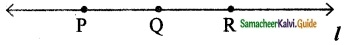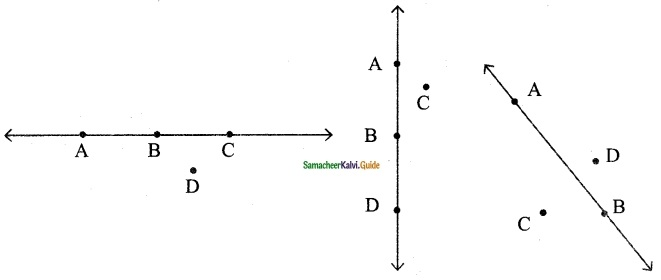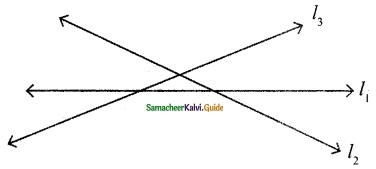Students can download Maths Chapter 4 Geometry Ex 4.3 Questions and Answers, Notes, Samacheer Kalvi 6th Maths Guide Pdf helps you to revise the complete Tamilnadu State Board New Syllabus, helps students complete homework assignments and to score high marks in board exams.

## Tamilnadu Samacheer Kalvi 6th Maths Solutions Term 1 Chapter 4 Geometry Ex 4.3

Question 1.
Observe the diagram and fill in the blanks.(i) ‘A’, ‘O’ and ‘B’ are ……… points.
(ii) ‘A’, ‘O’ and ‘C’ are ……….. points.
(iii) ‘A’,‘B’ and ‘C’are ……… points.
(iv) ……… is the point of concurrency.
Solution:
(i) collinear points
Hint: Points on a line.
(ii) non-collinear points
Hint: Points not on a line
(iii) endpoints/non-collinear points
(iv) O is the point of concurrency.
Hint: A points where lines meetQuestion 2.
Draw any line and mark any 3 points that are collinear.
Solution:Question 3.
Draw any line and mark any 4 points that are not collinear.
Solution:Question 4.
Draw any 3 lines to have a point of concurrency.
Solution:Question 5.
Draw any 3 lines that are not concurrent. Find the number of points of intersection.
Solution:Number of points of intersection = 3Objective Type Questions

Observe the Diagram and give answersQuestion 6.
A set of collinear points in the figure are
(a) A, B, C
(b) A, F, C
(c) B, C, D
(d) A, C, D
Solution:
(b) A, F, C
Hint: Collinear points are points on a line.Question 7.
A set of non-collinear points in the figure are ……….
(a) A, F, C
(b) B, F, D
(c) E, F, G
(d)A,D,C
Solution:
(d) A, D, C

Question 8.
A point of concurrency in the figure is ______
(a) E
(b) F
(c) G
(d) H
Solution:
(b) F